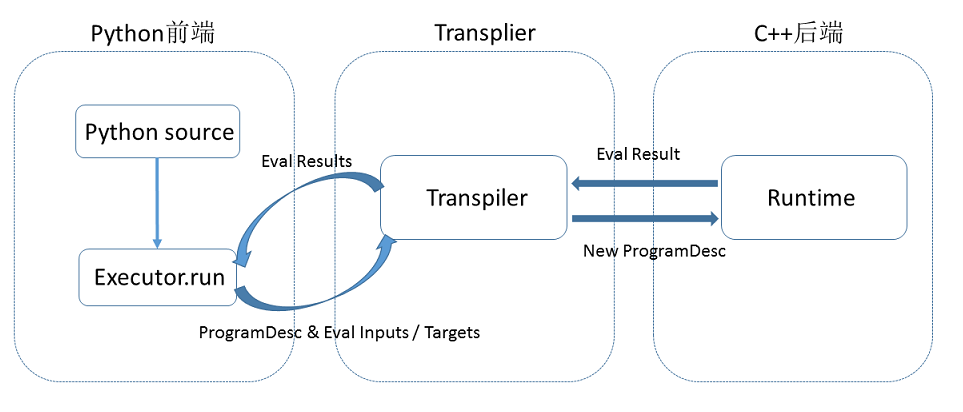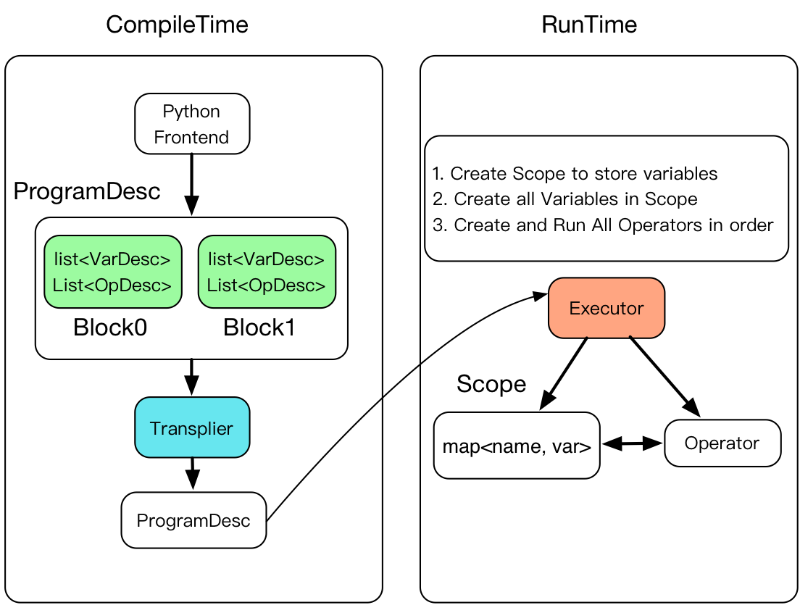# 设计思想¶

## 简介¶

• Program 如何描述模型

• Executor 如何执行运算

Paddle使用一种编译器式的执行流程，分为编译时和运行时两个部分，具体包括：编译器定义 Program ，创建Executor 运行 Program 。1. 编译时，用户编写一段python程序，通过调用 Paddle 提供的算子，向一段 Program 中添加变量（Tensor）以及对变量的操作（Operators 或者 Layers）。用户只需要描述核心的前向计算，不需要关心反向计算、分布式下以及异构设备下如何计算。

2. 原始的 Program 在框架内部转换为中间描述语言： `ProgramDesc`

3. `Transpiler` 接受一段 `ProgramDesc` ，输出一段变化后的 `ProgramDesc` ，作为后端 `Executor` 最终需要执行的 Program 。 `Transpiler` 并非必需步骤。

4. 执行 `ProgramDesc` 中定义的 Operator（可以类比为程序语言中的指令），在执行过程中会为 Operator 创建所需的输入输出并进行管理。

## 2. Program设计思想¶

```default_startup_program 可以由框架自动生成，使用时无需显式地创建

```
1. fluid.default_main_program ：定义了神经网络模型，前向反向计算，以及模型参数更新、优化器参数更新等各种操作。

```使用Paddle的核心就是构建起 default_main_program
```

### Programs and Blocks¶

Paddle 的 Program 的基本结构是一些嵌套 blocks，形式上类似一段 C++ 或 Java 程序。

blocks中包含：

• 本地变量的定义

• 一系列的operator

block的概念与通用程序一致，例如在下列这段C++代码中包含三个block：

```#include <iostream>

int main() {
int x = 5; // block 0
int y = 4; // block 0
int out;   // block 0

if (x < y) { // block 0
out = 1; // block 1
} else {
out = 0; // block 2
}

std::cout << out << std::endl;
return 0;
}
```

```import paddle.fluid as fluid

x = fluid.data(name='x', shape=, dtype='int64') # block 0
y = fluid.data(name='y', shape=, dtype='int64') # block 0

def true_block():
return fluid.layers.fill_constant(dtype='int64', value=1, shape=) # block 1

def false_block():
return fluid.layers.fill_constant(dtype='int64', value=0, shape=) # block 2

condition = fluid.layers.less_than(x, y) # block 0

out = fluid.layers.cond(condition, true_block, false_block) # block 0
```

### BlockDesc and ProgramDesc¶

`BlockDesc`中包含本地变量的定义 vars，和一系列的operator`ops`

```message BlockDesc {
required int32 idx = 1;
required int32 parent_idx = 2;
repeated VarDesc vars = 3;
repeated OpDesc ops = 4;
}
```

parent_idx表示父块，因此block中的操作符可以引用本地定义的变量，也可以引用祖先块中定义的变量。

Program 中的每层 block 都被压平并存储在数组中。blocks ID是这个数组中块的索引。

```message ProgramDesc {
repeated BlockDesc blocks = 1;
}
```

### 使用Blocks的Operator¶

Programs and Blocks的例子中，IfElseOp这个Operator包含了两个block——true分支和false分支。

```message OpDesc {
AttrDesc attrs = 1;
...
}
```

```message AttrDesc {
required string name = 1;

enum AttrType {
INT = 1,
STRING = 2,
...
BLOCK = ...
}
required AttrType type = 2;

optional int32 block = 10; // when type == BLOCK
...
}
```

## 3. Executor设计思想¶

Executor 在运行时将接受一个`ProgramDesc`、一个`block_id`和一个`Scope``ProgramDesc``block`的列表，每一项包含`block`中所有参数和`operator``protobuf`定义；`block_id`指定入口块；`Scope`是所有变量实例的容器。1. Executor 为每一个block创建一个Scope，Block是可嵌套的，因此Scope也是可嵌套的。

2. 创建所有Scope中的变量。

3. 创建并执行所有operator。

Executor的C++实现代码如下：

```class Executor{
public:
void Run(const ProgramDesc& pdesc,
Scope* scope,
int block_id) {
auto& block = pdesc.Block(block_id);

//创建所有变量
for (auto& var : block.AllVars())
scope->Var(Var->Name());
}

//创建OP并执行
for (auto& op_desc : block.AllOps()){
auto op = CreateOp(*op_desc);
op->Run(*local_scope, place_);
}
}；
```

```cpu=fluid.CPUPlace()
exe = fluid.Executor(cpu)
```

```...
x = numpy.random.random(size=(10, 1)).astype('float32')
outs = exe.run(
feed={'X': x},
fetch_list=[loss.name])
```

## 代码实例¶

```#加载函数库
import paddle.fluid as fluid #block 0
import numpy

#定义数据
train_data=numpy.array([[1.0],[2.0],[3.0],[4.0]]).astype('float32')
y_true = numpy.array([[2.0],[4.0],[6.0],[8.0]]).astype('float32')
#定义网络
x = fluid.data(name="x",shape=[None, 1],dtype='float32')
y = fluid.data(name="y",shape=[None, 1],dtype='float32')
y_predict = fluid.layers.fc(input=x,size=1,act=None)
#定义损失函数
cost = fluid.layers.square_error_cost(input=y_predict,label=y)
avg_cost = fluid.layers.mean(cost)
#定义优化方法
sgd_optimizer = fluid.optimizer.SGD(learning_rate=0.01)
sgd_optimizer.minimize(avg_cost)
```

```print(fluid.default_main_program().to_string(True))
```

```blocks {
idx: 0
parent_idx: -1
vars {
name: "mean_1.tmp_0"
type {
type: LOD_TENSOR
lod_tensor {
tensor {
data_type: FP32
dims: 1
}
}
}
persistable: false
}
vars {
name: "square_error_cost_1.tmp_1"
type {
type: LOD_TENSOR
lod_tensor {
tensor {
data_type: FP32
dims: -1
dims: 1
}
lod_level: 0
}
}
persistable: false
}
vars {
name: "square_error_cost_1.tmp_0"
type {
type: LOD_TENSOR
lod_tensor {
tensor {
data_type: FP32
dims: -1
dims: 1
}
lod_level: 0
}
}
persistable: false
...
```

BlockDesc中包含定义的 vars 和一系列的 ops，以输入x为例，python代码中定义 x 是一个数据类型为”float32”的1维数据：

```x = fluid.data(name="x",shape=[None, 1],dtype='float32')
```

```vars {
name: "x"
type {
type: LOD_TENSOR
lod_tensor {
tensor {
data_type: FP32
dims: -1
dims: 1
}
lod_level: 0
}
}
persistable: false
```

dims表示数据的维度，这里表示 x 的维度为[-1,1]，其中-1是batch的维度，无法确定具体数值时，Paddle 自动用 -1 占位。

``` #在CPU内执行训练
cpu = fluid.CPUPlace()
#创建Executor
exe = fluid.Executor(cpu)
```

``` #参数初始化
exe.run(fluid.default_startup_program())
```

```#开始训练
outs = exe.run(
feed={'x':train_data,'y':y_true},
fetch_list=[y_predict.name,avg_cost.name])
```

```[array([[1.5248038],
[3.0496075],
[4.5744114],
[6.099215 ]], dtype=float32), array([1.6935859], dtype=float32)]
```